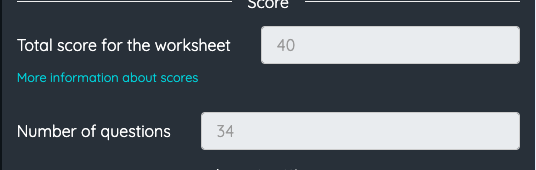Go back

# scoring issue

SOLVED

U5B Post-test (this is our first try giving a math test using Topworksheet)How is the total score for worksheet 40? I keep counting 33 - I have tried a couple different ways.

How is this worksheet 34 questions? I count 27 questions with points. (there are 34 boxes but some are not score)

* can the points be half points - for instance in the multiple choice....

A:  0pts          B: 0.5 pt       C:  0 pts        D: 1 pt

* for formula question/answers - even if the question is 1 point. - we would like the option to give students 0.5.

I see in the drawing box with pts - you gave us a combo box for pt options, but sometimes we give 2.5 points.

04/19/2023Christian TopWorksheets 04/19/2023